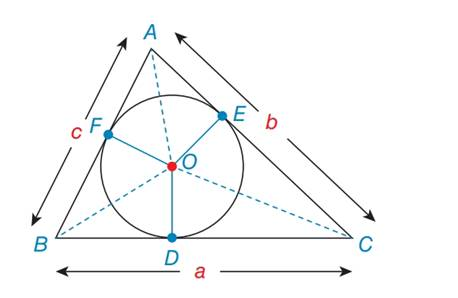Chapter 7.2, Problem 45EElementary Geometry For College St...

7th Edition
Alexander + 2 others
ISBN: 9781337614085

Solutions

Chapter
SectionElementary Geometry For College St...

7th Edition
Alexander + 2 others
ISBN: 9781337614085
Textbook Problem

For exercises 44 to 46, O is the inscribed circle for ∆ A B C . In exercises 45 and 46, use the result from 44.Explain why ∠ F O A         and         ∠ B O C are supplementary.To determine

To explain:

Why FOAandBOC are supplementary.

Explanation

Definition:

Supplementary Angle:

When the two angle add to 180, we say they supplement each other.

Given:

Explain FOAandBOC are supplementary.

Calculation:

By the given incircle triangle,

O is the 180 because the angle is a straight line and a straight line measures a 180

Still sussing out bartleby?

Check out a sample textbook solution.

See a sample solution

The Solution to Your Study Problems

Bartleby provides explanations to thousands of textbook problems written by our experts, many with advanced degrees!

Get Started

Convert the expressions in Exercises 6584 to power form. 35x5x8+72x3

Finite Mathematics and Applied Calculus (MindTap Course List)

Subtract and check: 40001180_

Elementary Technical Mathematics

In Exercises 3538, find the transpose of each matrix. 

Finite Mathematics for the Managerial, Life, and Social Sciences

Evaluate . e + 1

Study Guide for Stewart's Single Variable Calculus: Early Transcendentals, 8th